## Sunday, November 25, 2012

### Two more calculators added to the collection.

I love swap meets and pawn shops. Eddie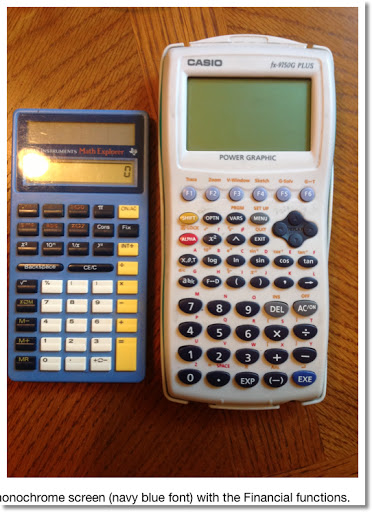### f(x) = x * random ^ random

Earlier this month, I received a request from Jason Foose. These are six graphs of y = x * rand^rand: two with the TI-84+, two with the Casio Prizm (fx-CG 10, and two with the HP 39gii.

On the Prizm, I had to use the catalog to find the random number function (labeled Ran#).

Re-editing the function will cause the function to be redrawn.

Enjoy!

Eddie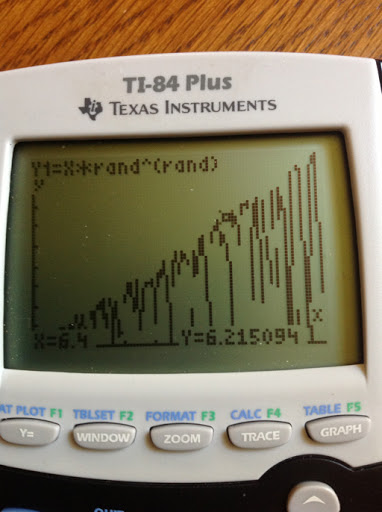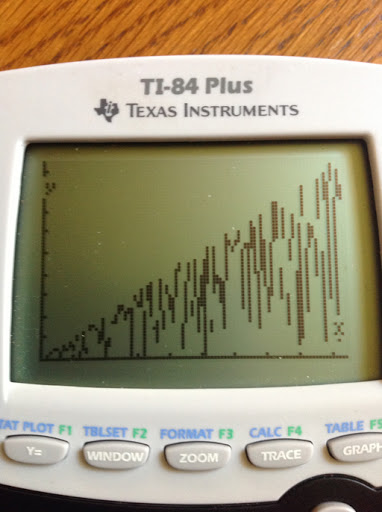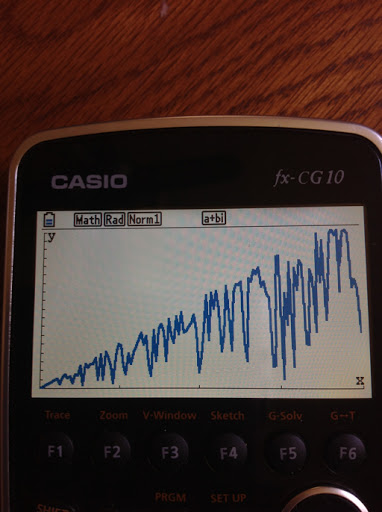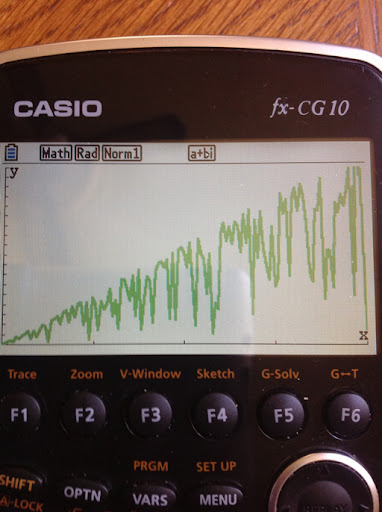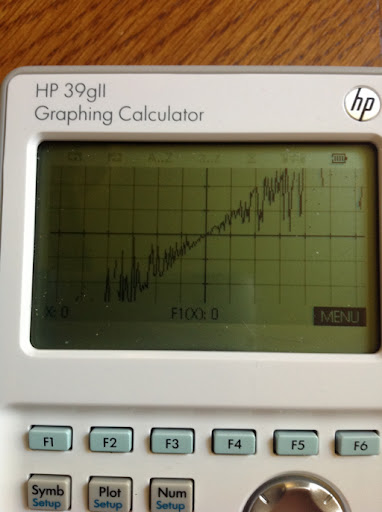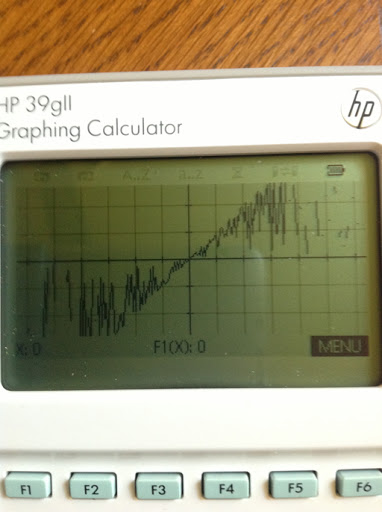### Matrix Functions on the fx-991ES (fx-115ES in the US) vs TI-36X Pro

Matrix Function Comparison (fx-991ES/fx-115ES vs TI 36X Pro)

Today's blog entry comes from a question by An Artist:

An Artist has left a new comment on your post "TI-36X Pro Review":

Hi Eddie,

In India, the TI 36x pro is available at the same price as the Casio 991ES.
I have ordered the TI.

Is this a good decision?
I've heard of a memory overload bug of the TI which gives wrong answers. Can you please tell me how to get around the bug?

My main focus is matrix and someone told me that this one has more matrix functions for matrices. Can you please confirm that?

First of all thank you for the compliment An Artist, very much appreciated.

Regarding the overload bug on the TI-36X Pro, honestly this is the first time I read of it. According to one of the reviewers on the flipkart.com page, the reviewer reports an overload bug occurs when too many equations are used or too much memory is used. I have not found any additional information about this. Personally I have not encounter this problem.

Now the matrix commands. I am going to list the available matrix functions for each calculator. First up, Casio, then Texas Instruments.

Keep in mind both calculators allow only for real-numbered elements.

Casio fx-991ES (aka fx-115ES in the United States)

You will need to enter a specific mode for Matrices (MODE 6)

3 matrices can be stored, up to size 3 x 3 matrices. A separate "Ans" matrix is also available.

Matrix Functions:
Arithmetic
Ability to resize matrices
Determinant (dim)
Transpose (Trn)
Inverse (with the x⁻¹ button)
Square of a square matrix (with the x² button)
Cube of a square matrix (with the x³ function)
Absolute Value of each element (Abs)

Matrices are automatically cleared when modes are switched.

Note: The updated fx-115ES PLUS adds the ref and rref functions.

Texas Instruments TI-36X Pro

There is no specific mode required, you can work with matrices directly from the Home screen.

3 matrices can be stored, up to size 3 x 3 matrices. A separate "Ans" matrix is also available. The TI-36X offers identity matrices [I2] and [I3] of size 2 x 2 and 3 x 3, respectively.

Matrix Functions:
Arithmetic
Ability to resize matrices (through the edit screen)
Determinant (dim)
Transpose (Trn)
Inverse
Square of a square matrix (with the x² button)
Powers of a square matrix ([A]^n where n is a positive integer, 0, or -1)
Absolute Value of each element (abs)
Integer Part of each element (iPart)
Fractional Part of each element (fPart))
Rounding of each element (round)
Reduced Echelon Form (ref)
Row Reduced Echelon Form (rref)

Matrices are retained in memory on the TI-36X Pro.

An Artist, to answer your question between the fx-991ES and the TI-36X Pro, I can confirm that the TI-36X Pro has more functions for matrices. Hope this helps,

Eddie

## Friday, November 23, 2012

### My next blog series?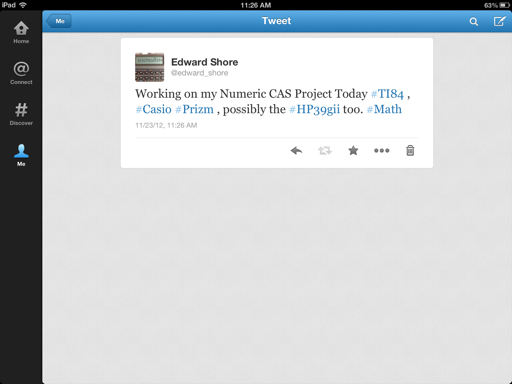## Thursday, November 22, 2012

### Happy Thanksgiving

Wishing everyone a happy and safe Thanksgiving. I am very thankful to all who follow my blog and all who read and make comments. You are truly the best!

May Thanksgiving be a blessed event, memorable, and stress be minimized!

Eddie

## Sunday, November 18, 2012

### Graphing Calculator Programming Languages Comparison: TI 84 Plus vs Casio Prizm

This is a short comparison between the programming languages of the Texas Instruments TI-84+ and the Casio Prizm. For each category, I will list the different syntaxes required.

If you find a program that you like that is programmed on a calculator you don't have, no fear! This guide can be served as a translation guide. For more details, consult the manual or search for detailed tutorials.

Hope this helps,

Eddie

Notes:

The commands for the TI-84+ also covers the TI-84+ Silver Edition, TI-83+ Silver Edition, TI-83+, and most likely the TI-82 and the upcoming TI-84+ Plus C Silver Edition (84+ with a color screen). The TI-84+ family carries a program memory of about 24,000 bytes, 28,000 for the TI-82.

The commands for the Casio Prizm (Model fx-CG 10/20) also apply to the fx-9860g (all versions), fx-9750g, and (most likely) fx-9850g. The Casio family carriers about 60,000-62,000 bytes of program memory, except the 9850g (32,000 bytes).

Keystrokes may vary.

Arguments in each command are in italics.

Here we go:

Numerical Derivative

TI-84+: MATH, 8
nDeriv(f(variable),var,value)

Casio Prizm: OPTN, F4, F2
d/dx(f(X),value)
The variable is always X.

Definite Integral

TI-84+: MATH, 9
fnInt(f(var), var, lower limit, upper limit)

Casio Prizm: OPTN, F4, F4
∫(f(X),lower limit,upper limit)
The variable is always X.

Solve

TI-84+: Catalog or MATH, B in programming mode
solve(expression,variable to be solved for,guess, range*)
expression is set to be equal to 0
range is a two element list {low, high}, and is an optional argument.

Casio Prizm:

There are two commands.

Solve f(X)=0 for X: OPTN, F4, F1
Solve(f(X),guess,low,high)

Solve an equation in any variable: OPTN, F4, F5
SolveN(equation,variable to be solved for,low,high)

Sums

TI-84+: MATH, 0
Σ(f(var),var,start value,end value)

Casio Prizm: OPTN, F4, F6, F3
Σ(f(var),var,start value,end value)

TI-84+:
Input "prompt string", var

Casio Prizm:
"prompt string"? → var

Displaying Results

TI-84+:
Disp var or string
You can add other lines, using commas to separate them.

Pause var
Pause allows the user to scroll the variable's value.

Casio Prizm:
var or string
The ◢ is the right triangle symbol, which acts like a pause command.

If Then Else

TI-84+:
If test expression
Then
do this if test is true
Else
do this if test is false
End

Casio Prizm:
If test expression
Then
do this if test is true
Else
do this if test is false
EndIf

Shortcut: Jump Command
test condition1 command if test is true : skip to here if the test is false

For Loop

TI-84+:
For(counter var,start value,end value,step size*)
commands
End

step size can be positive or negative, and is optional

Casio Prizm:
For start valuevar To end value Step step size*
commands
End

step size can be positive or negative, and is optional

While Loop

TI-84+:
While this test condition is true
do these commands
End

Casio Prizm:
While this test condition is true
do these commands
WhileEnd

Do Until Loop

TI-84+:
Repeat until this condition becomes true
these commands
End

Casio Prizm:
Do
these commands
LpWhile this condition remains false

List and Matrix Element Calls

TI-84+:
Lists:
L#(element number)
#: 1 through 6 or custom name

Matrix:
[[#]](row,column)
#: A through J

Casio Prizm:
Lists:
List #[element number]
#: 1 through 26 or custom name

Matrix:
Mat #[row,column]
#: 1 through 26

Giving the user a menu of options

TI-84+:

Casio Prizm:
Menu "title string", "choice 1", label name...

Decrementing and Incrementing Variables by 1

TI-84+:
IS>(var,target value)
do if var + 1 ≤ target value

DS<(var,target value)
do if var - 1 ≥ target value

Casio Prizm:
ISZ var
do if var + 1 ≠ 0

DSZ var
do if var - 1 ≠ 0

This blog is property of Edward Shore, 2012.

## Sunday, November 11, 2012

### Jacobi Elliptical Functions

This blog entry is possible thanks to @mathematicsprof on Twitter. He posted an article "Jacobi Elliptic Functions from a Dynamic Systems Point of View", written by Ken Meyer Ph.D of the University of Cincinnati, which not only gave me something to read during lunch last Friday but also lead me on how to calculate Jacobi Elliptic Functions. I am so grateful!

By the way, I am on Twitter: @edward_shore.

Jacobi Elliptic Functions - An Introduction

Let k and t be parameters where 0 < k < 1 as the following system of differential equations is to be solved:

dx/dt = y(t) * z(t)
dy/dt = -z(t) * x(t)
dz/dt = -k^2 * x(t) * y(t)

which satisfy the initial conditions x(0) = 0, y(0) = 1, and z(0) = 1.

The Jacobi Elliptical Functions are defined to be the solutions to the above system.

The sine amplitude function is defined as sn(t, k) = x(t).

The cosine amplitude function is defined as cn(t, k) = y(t).

The delta amplitude function is defined as dn(t,k) = z(t).

A very interesting point, which has also managed to trip me up for years, is that there is no explicit closed formula for sn(t,k), cn(t,k), and dn(t,k). Yet mathematicians can find the derivatives and integrals of these functions.

Derivatives of the Jacobi Elliptical Functions

These derivatives, by looking at the system above, are:

d/dt sn(t,k) = cn(t,k) * dn(t,k)
d/dt cn(t,k) = -dn(t,k) * sn(t,k)
d/dt dn(t,k) = -k^2 * sn(t,k) * cn(t,k)

When k=0

By setting k=0 and finding solutions to the system equations becomes:

dz/dt = 0
z(t) = c
Since z(0)=1, conclude z(t)=1.

Then
dx/dt = y(t)
dy/dt = -x(t)
with initial conditions x(0)=0 and y(0)=1.

Since sin(0)=0, cos(0)=1, d/dt sin t = cos t, and d/dt cos t = -sin t, we can conclude that x(t) = sin t and y(t) = cos t. Meyer states that as k approaches 0, sn(t,k) approaches sin(t), cn(t,k) approaches cos(t), and dn(t,k) approaches 1.

A Very Familiar Identity

Similar to the famous identity:

sin²(θ) + cos²(θ) = 1

An identity of Jacobi Elliptical functions is:

sn²(t,k) + cn²(t,k) = 1

Why is this true? Take the derivative with respect to t and we find that:

2 sn(t,k) cn(t,k) dn(t,k) - 2 cn(t,k) dn(t,k) sn(t,k) = 0

How to Calculate

We can use the integral definition to assist us in calculating values for sn, cn, and dn. In this section, let the parameters be u and k, respectively. The integral definition stems from the incomplete elliptical integral: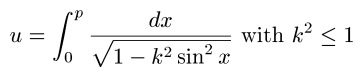where u and k are known and p is the value we are solving for.

The value p is known as the Jacobi Amplitude, am(u,k) for short. Then:

am(u,k) = p

sn(u,k) = sin(am(u,k)) = p

cn(u,k) = cos(am(u,k)) = p

dn(u,k) = √(1 - k² sin² (am(u,k)) = √(1 - k² sin²(p))

Advanced mathematics software or an online calculator, such as this one from Ke!san, are often used to calculate values of Jacobi Elliptical Functions. Fortunately, this task can be done with high-end graphing calculators, using their solve application.

I have been able to obtain accurate answers using the Hewlett Packard HP-50g, Texas Instruments TI-84+, and Casio Prizm fx-CG 10. The later two are relatively fast in finding values. Below I will show the setup for each of the three calculators. I am pretty sure the TI nSpire can handle this too. The following screens show how to obtain am(u,k), the Jacobi Elliptical functions easily follow.

In the solver screen, you can leave X blank (HP-50G only), or just assign any arbitrary value to X, such as 1, since X is the dummy variable in the integration and has no effect on calculations.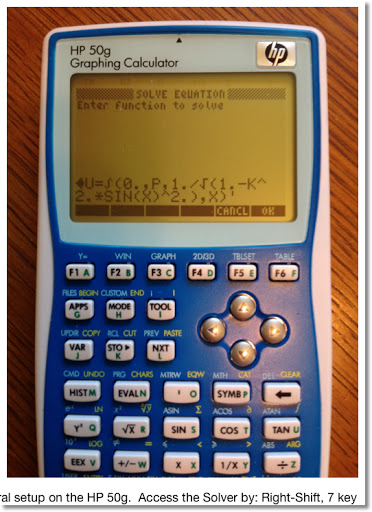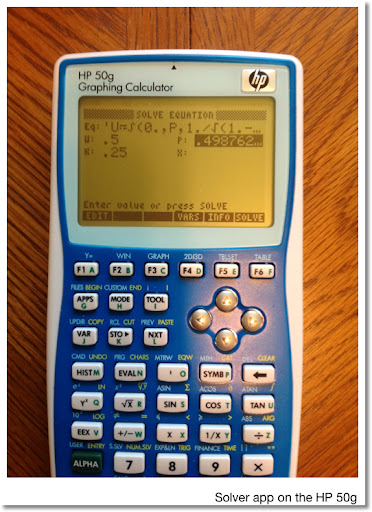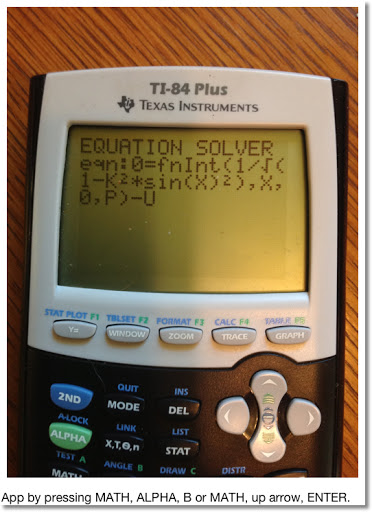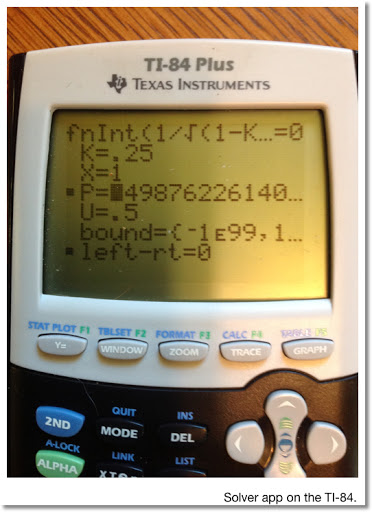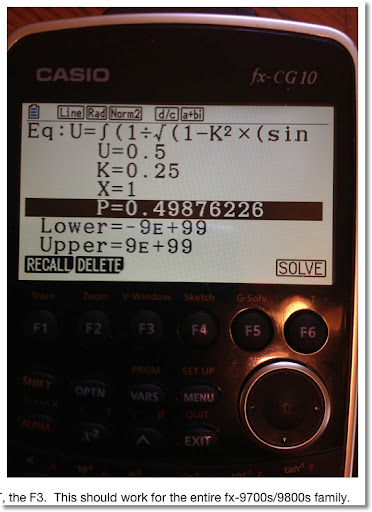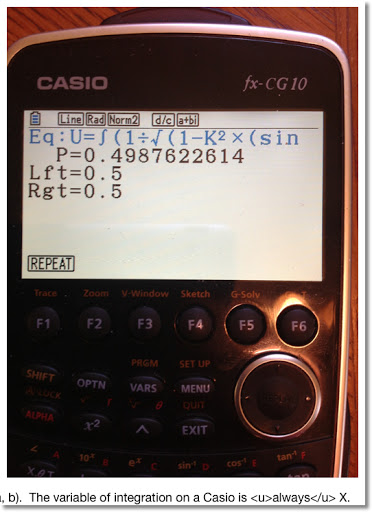Here is a small table of values: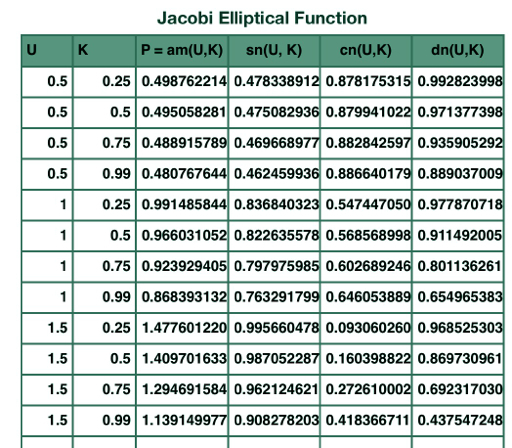Resources

Meyer, Kenneth R. "Jacobi Elliptic Functions from a Dynamic Systems Point of View" The Mathematical Association of America. Monthly 108. October 2001 - retrieved 11/9/2012

Weinstein, Eric "Jacobi Elliptic Functions" - From MathWorld - A Wolfram Web Source, http://mathworld.wolfram.com/JacobiEllipticFunctions.html, retrieved 11/11/2012

Fun as always! Thank you as always. Until next time, Eddie

This blog is property of Edward Shore, 2012.

### The United States Fiscal Cliff: What does it all mean?

Hi everybody. Today I want to talk about tax policy and how the potential tax changes come January 1, 2013 affects taxpayers in the United States.

When the United States Congress goes back into session, one of the hot topics will be the "fiscal cliff". The "fiscal cliff" is a set of income tax credits and tax rates that are set to expire on January 1, 2013; effectively raising the income tax liability for each taxpayer. This blog entry will show how much of a tax increase to expect if no action is taken.

CAUTION AND DISCLAIMER:

1. The information presented today is for general purposes only and is not to be used as income tax advice. If you need advice, please work with a licensed income tax professional.

2. I am only working with federal income tax - be aware that other taxes can increase and decrease.

3. There is no guarantee of the final tax rates for 2013.

4. For today's blog, I will work with single and married filers (married filing jointly). Taxpayers that are head of households, married but filing separately, and other statuses may have different tax rates.

Current United States Tax Law for 2012

These are the current tax laws. When Americans file their income tax early next year, they will calculate tax liability using these rates.

2012 Tax Rates

(income range, tax rate)

Single
\$0-\$8,700; 10% of income
\$8,700-\$35,350; \$870 plus 15% of income excess of \$8,700
\$35,350-\$85,650; \$4,867.50 plus 25% of income excess of \$35,350
\$85,650-\$178,650; \$17,442.50 plus 28% of income excess of \$85,560
\$178,650-\$388,350; \$43,482.50 plus 33% of income excess of \$178,650
\$388,350 and above; \$112,683.50 plus 35% of income excess of \$388,350

Standard Deduction: \$5,950 (phased out as income passes a certain point)

Married Filing Jointly (filing as a couple)
\$0-\$17,400; 10% of income
\$17,400-\$70,700; \$1,740 plus 15% of income excess of \$17,400
\$70,700-\$142,700; \$9,735 plus 25% of income excess of \$70,700
\$142,700-\$217,450; \$27,735 plus 28% of income excess of \$142,700
\$217,450-\$388,350; \$48,665 plus 33% of income excess of \$217,450
\$388,350 and above; \$105,062 plus 35% of income excess of \$388,350

Standard Deduction: \$11,900 (phased out as income passes a certain point)

Personal Exemption: \$3,800 (almost every person gets one)

Let's take an example for a single person in the United States who earned \$50,000. Every taxpayer gets a personal exemption, and assume that this person takes a standard deduction in lieu of itemized deductions. Assuming no other credits apply:

Income: 50,000 - 3,800 - 5,950 = 40,250
Tax Liability for 2012: 4,867.50 + (40,250 - 35,350) * 25% = \$6,092.50

Tax Rates for 2013 if No Action is Taken

If no action is taken, the income tax rates for each bracket will rise, except for the 15% bracket. The 10% bracket disappears entirely. The amounts shown in the table are 2012 amounts to determine the tax brackets - which will most likely be adjusted for inflation. These are estimated tables only, not final.

Tax Increase in 2013?

(income range, tax rage)

Single

\$0-\$35,350; 15% of income
\$35,350-\$85,650; \$5,302.50 plus 28% of income excess of \$35,350
\$85,650-\$178,650; \$19,386.50 plus 31% of income excess of \$85,650
\$178,650-\$388,350; \$48,216.50 plus 36% of income excess of \$178,650
\$388,350 and above; \$123,708.50 plus 39.6% of income excess of \$388,350

Standard Deduction: \$5,950 but will probably increase - final number not released

Married Filing Jointly

\$0-\$70,700; 15% of income
\$70,700-\$142,700; \$10,605 plus 28% of income excess of \$70,700
\$142,700-\$217,450; \$30,765 plus 31% of income excess of \$142,700
\$217,450-\$388,350; \$53,937.50 plus 36% of income excess of \$217,450
\$388,350 and above; \$115,461.50 plus 39.6% of income excess of \$388,350

Standard Deduction: \$9,900 - due to the "marriage penalty"

Personal Exemption: \$3,800 but will most likely increase due to inflation

Let's take the same person, who is Single and makes \$50,000. Assume income level stays level for 2013 and the person takes the standard deduction. Then for 2013:

Income: \$50,000 - \$5,950 - \$3,800 = \$40,250
Estimated Tax Liability for 2013: \$5,302.50 + (\$40,250 - \$35,350) * 28% = \$6,674.50

This represents a potential increase of \$582 in income taxes.

You can use these estimate these tax brackets to estimate the increase in federal income taxes in 2013 - should nothing happen and the Bush Tax Brackets, which were set back in 2001 and 2003, expire.

In addition, the potential tax laws can change in 2013:

* An increase in long term capital gain tax, from 15% to 20%. This tax is for profit made on selling stocks and other long term capital assets held for more than 1 year.
* Social security tax on wages increase from 4.2% to 6.2%. Personally, I see this happening regardless of what happens in Congress over the next two months.
* The exclusion of employer-provided education assistance of \$5,250 can disappear in 2013.
* The American Opportunity Tax Credit revers to the Hope Credit. The credit would be reduced from \$2,500 to \$1,800 and will only be available to students in the first two years of undergraduate education instead of four.
* The Child Credit and Earned Income Tax Credit is set to take a hit for 2013.

Hopefully this will alleviate some fear, or at the very least prepare taxpayers for what could be coming.

What is Congress Discussing

There is talk about extending the Bush tax brackets for at least another year for most taxpayers. Those with the highest income tax brackets (33% and 35%) could see their income tax increase and that what is in debate. Hopefully soon, Americans will know the final income tax structure for 2013

Source:
Discussion by Godfrey Kahn S.C., 5/23/2012 - Retrieved 11/11/2012

Good day everyone. This blog is information purposes only and not to start political debate.

Eddie

## Saturday, November 10, 2012

### Bernoulli Numbers and Polynomials

Today is a good day. I went to the library of alma mater, Cal Poly Pomona for library day. Once a month I try to visit a university library and peruse through with Mathematics Section.I finally got the concept of generating functions. In the many years I studied math, generating functions proved to be an elusive topic for me. Not any more.

What are generating functions?

Generating Functions

Generating functions is a power series. The series is usually does not terminate. The coefficients of the power series can reveal a sequence used in various fields in science.

The Ordinary Generating Function:

G(a_n; x^n) = ∑ a_n * x^n

Sometimes we have an Exponential Generating Function:

E(a_n; x^n) = ∑ a_n * (x^n/n!)

There are other types of generating functions, but I will focus on the basic types described above.

n! represents the factorial of n. In this case, n is a positive integer, and:

n! = n * (n-1) * (n-2) * ... * 3 * 2 * 1

By definition, 0! = 1

To expand the generating function G(a_n; x^n), calculate a Maclaurin Series of G(x). A Maclaurin Series of a function is a Taylor Series about the point x = 0. Hence the Maclaurin Series:

G(x) = G(0) + G'(0) * x/1! + G''(0) * x^2/2! + G'''(0) * x^3/3! + .... + O(x)

where O(x) is the error term G^(n+1)(z) * x^(n+1)/(n+1)! In practice and calculation, O(x) is sometimes "ignored".

An Example: A Basic Generating Function

Expand the generating function, let's go five terms:

G(a_n; x^n) = 1/(1 - x)

G(x) = 1/(1 - x)
Then:
G(0) = 1/(1 - 0) = 1

dG/dx = 1/(x-1)^2; dG/dx(0) = 1

d^2G/dx^2 = -2/(x-1)^3; d^2G/dx^2(0) = 2

d^3G/dx^3 = 6/(x-1)^4; d^3G/dx^3(0) = 6

d^4G/dx^4 = -24/(x-1)^5; d^4G/dx^4(0) = 24

To five terms...

1/(1-x) = 1 + 1 * x/1! + 2 * x^2/2! + 6 *x^3/3! + 24 * x^4/4! + O(x)
= 1 + x + x^2 + x^3 + x^4 + O(x)

The sequence of coefficients are: {1, 1, 1, 1, 1}.

The Bernoulli Numbers

The Bernoulli Numbers can be found by using the Exponential Generating Function:

E(a_n; x) = ∑ x/(e^x - 1)

The Bernoulli Numbers, B_n, are the "coefficients" of the expansion of ∑ x/(e^x - 1). Recall in the Exponential Generating Function, the "coefficients" are of the term x^n/n!.

With E(x) = x/(e^x - 1), the first two derivatives are:

dE/dx = - ((x-1)*e^x + 1)/(e^(2x) - 2*e^x + 1)

d^2E/dx^2 =
((x - 2)*e^(2x) + (x + 2)*e^x) / (e^(3x) - 3*e^(2x) + 3*e^(2x) - 1)

Note that calculating E(0) gives us 0/0. Same for dE/dx(0) and d^2E/dx^2(0). However, if I use a calculate with CAS capabilities such as the Hewlett Packard HP 50g, or mathematical software such as MathStudio (an app for smartphones and iPads), I get something like this (first eight terms):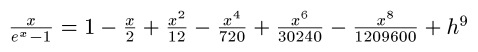Why is that?

Observe that:

lim x/(e^x - 1) as x → 0 = 0/0

Using the L'Hospital's rule, we can take the derivatives of both numerator and denominator,

lim 1/(e^x) as x → 1 = 1

which implies that:

lim x/(e^x - 1) as x → 0 = 1

You can generate terms by taking the limit as x → 0 for each term.

This how we end up with the series. Now to extract the "coefficients", observe that:

12 = 6 * 2!
(no term contains x^3/3!)
720 = 30 * 4!
(no term contains x^5/5!)
30240 = 42 * 6!
(no term contains x^7/7!)
1,209,600 = 30 * 8!

Our sequence for this generating function (for nine terms) is:

{1, -1/2, 1/6, 0, -1/30, 0, 1/42, 0, -1/30}

These numbers are the Bernoulli numbers. In fact, definition by generating function is:

x/(e^x - 1) = ∑ B_n * (x^n/n!)

Bernoulli Numbers

B_0 = 1
B_1 = -1
B_2 = 1/6
B_3 = 0
B_4 = -1/30
B_6 = 1/42
B_8 = -1/30
B_10 = 5/66
B_12 = -691/2730
B_14 = 7/6

B_n = 0 where n is odd and n > 2

Bernoulli Polynomials

Bernoulli Polynomials can be generated by the following formula:

β_n(x) = ∑((B_k * n!/(k!*(n-k)!) * x^k, from k = 0 to n)

where B_k is the kth Bernoulli number.

Source:
Krylov, Vladimir Ivanoch, translated by H. Stroud Approximate Calculations of Integrals McMillian Company: New York, 1962

Until next time, be safe everyone! Eddie

This blog is property of Edward Shore, 2012.

## Friday, November 9, 2012

### The Sine Wave - A Portrait

Good Friday!

One of my favorite mathematical functions is the sine function. Not only the sine wave helps describe a lot of physical phenomena, but I also see it like how life goes, with its ups and downs.

So here are few graphs (portraits) of the sine wave.

Settings:
Calculator: TI-84+
Window: X range: -π/2 to 5π/2, Y range: -2.05 to 2.05
(Except the last one, X range: 0 to 5π/2)

Eddie

This blog is property of Edward Shore, 2012.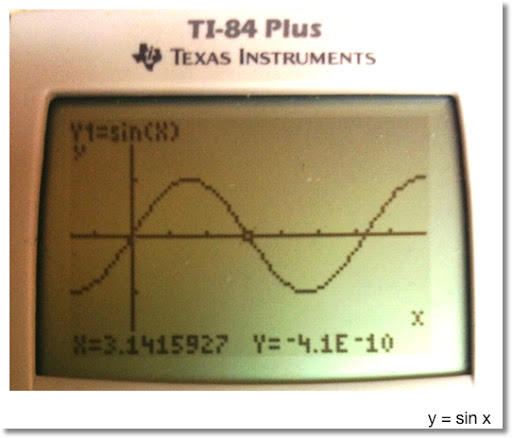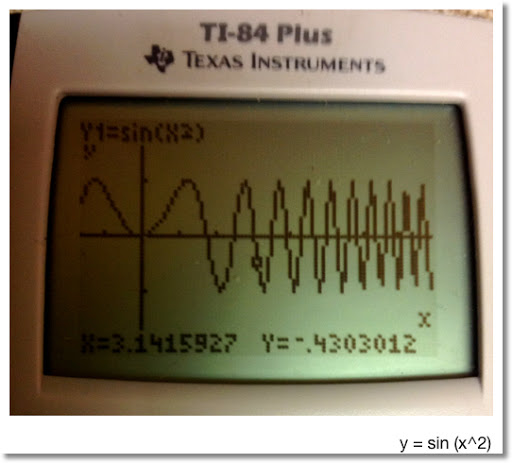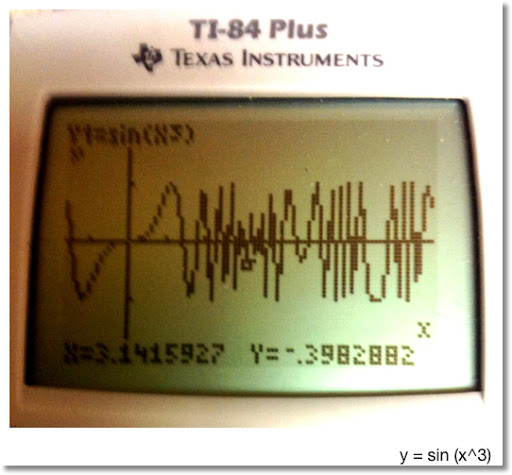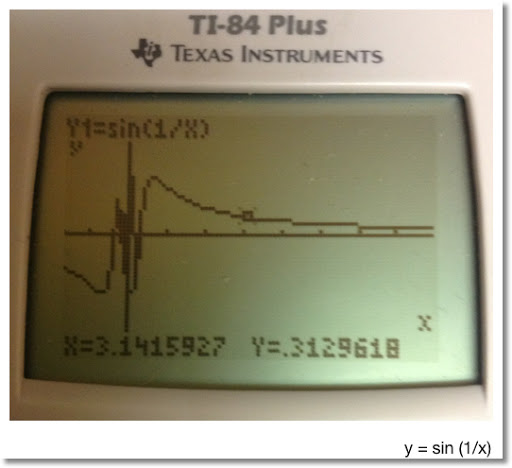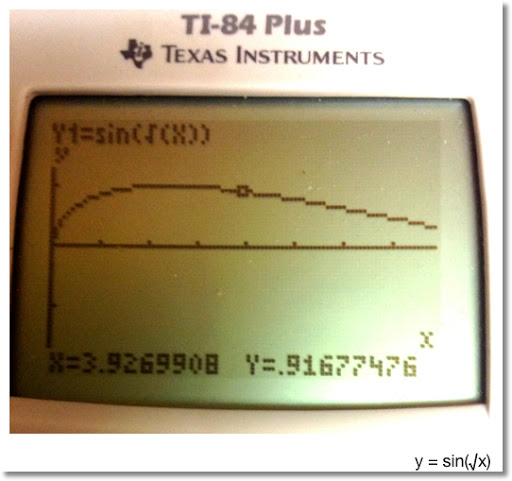### HP 15C: Error Function and Lower Tail Normal Cumulative Function

HP 15C:   Error Function and Lower Tail Normal Cumulative Function Formulas Used  Error Function  erf(x) = 2 ÷ √π * ∫( e^(-t^2) dt, t = 0 t...